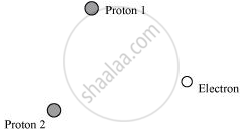# If one of the two electrons of a H2 molecule is removed, we get a hydrogen molecular ion HH2+. In the ground state of an HH2+, the two protons are separated - Physics

Numerical

If one of the two electrons of a Hmolecule is removed, we get a hydrogen molecular ion "H"_2^+. In the ground state of an "H"_2^+, the two protons are separated by roughly 1.5 Å, and the electron is roughly 1 Å from each proton. Determine the potential energy of the system. Specify your choice of zero potential energy.

#### Solution

The system of two protons and one electron is represented in the given figure.Charge on proton 1, q1 = 1.6 × 10−19 C

Charge on proton 2, q2 = 1.6 × 10−19 C

Charge on electron, q3 = −1.6 × 10−19 C

Distance between protons 1 and 2, d1 = 1.5 × 10−10 m

Distance between proton 1 and electron, d2 = 1 × 10−10 m

Distance between proton 2 and electron, d3 = 1 × 10−10 m

The potential energy at infinity is zero.

Potential energy of the system,

"V" = ("q"_1"q"_2)/(4piin_0"d"_1) + ("q"_2"q"_3)/(4piin_0"d"_3) + ("q"_3"q"_1)/(4piin_0"d"_2)

Substituting 1/(4piin_0) = 9 xx 10^9  "N m"^2   "C"^-2, we obtain

"V" = (9 xx 10^9 xx 10^-19 xx 10^-19)/(10^-10) [-(16)^2 + (1.6)^2/(1.5) + -(1.6)^2]

= -30.7 xx 10^-19  "J"

= −19.2 eV

Therefore, the potential energy of the system is −19.2 eV.

Concept: Potential Energy of a System of Charges
Is there an error in this question or solution?
Chapter 2: Electrostatic Potential and Capacitance - Exercise [Page 88]

#### APPEARS IN

NCERT Physics Part 1 and 2 Class 12
Chapter 2 Electrostatic Potential and Capacitance
Exercise | Q 2.19 | Page 88
NCERT Class 12 Physics Textbook
Chapter 2 Electrostatic Potential and Capacitance
Exercise | Q 19 | Page 90
Share